Courses

WBJEE Chemistry Test - 6

40 Questions MCQ Test WBJEE Sample Papers, Section Wise & Full Mock Tests | WBJEE Chemistry Test - 6

Description
Attempt WBJEE Chemistry Test - 6 | 40 questions in 60 minutes | Mock test for JEE preparation | Free important questions MCQ to study WBJEE Sample Papers, Section Wise & Full Mock Tests for JEE Exam | Download free PDF with solutions
QUESTION: 1

NH₄Cl in liquid NH₃ will act as a/an :

Solution: The reaction between NH3 (weak base) and HCl (strong acid) forms NH4Cl. NH4+ acts as a weak acid and Cl- does not act like a base, same as NO3-. This means the salt will be weakly acidic.
QUESTION: 2

Solution:
QUESTION: 3

Fe(OH)₃ can be separated from Al(OH)₃ by the addition of

Solution:
QUESTION: 4
Which of the following does not turn Schiff's reagent to pink ?
Solution:
QUESTION: 5
Sometimes yellow turbidity appears while passing H₂S gas even in the absence of II group radicals. This is because of
Solution:
QUESTION: 6

The energy of second Bohr orbit of the hydrogen atom is -328KJ mol⁻1. The energy of fourth Bohr orbit would be

Solution:
QUESTION: 7
On hydrolysis of starch, we finally get
Solution:
QUESTION: 8

The heat of formation of CO₂ is -94 Kcal mol⁻1 whereas ∆H for 2CeO₂ → 2CO is -110.5 Kcal,The enthalpy of combustion carbon is

Solution:
QUESTION: 9

Among the molecules (a)XeO₃ (b)XeOF (c)XeOF₆,those having same no. of lone pairs on Xe are

Solution: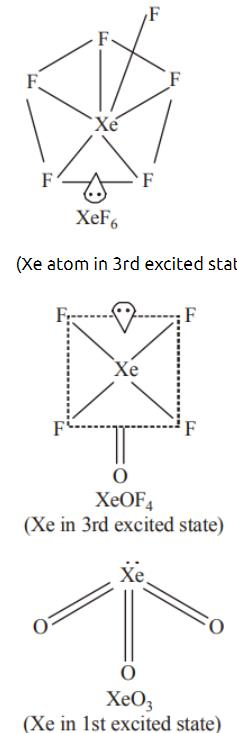Thus, all the three have same no. of lone pair of electrons i.e. one.

QUESTION: 10

The equilibrium constant for the following reaction will be 3 A + 2 B → C

Solution:
QUESTION: 11
The most reactive nucleophile among the following is
Solution: Although due to +I effect of methyl groups, (CH3)2 CHO and (CH3)3 CO are stronger bases but due to steric hindrance both are weaker nucleophiles than CH3O
Further due to resonance, C6H5O is the weakest nucleophile
CH3O is most reactive (stronger) nucleophile
QUESTION: 12

A reaction with respect to the reactant A has a rate constant of 5 s⁻1. If we start with[A]= 10 mol-L⁻1, then in what time the concentration of A becomes 1.0 mol-L⁻1?

Solution:
QUESTION: 13

In an electrochemical cell, if Eº is the EMF of the cell involving 'n' mole of electrons, then ∆Gº is

Solution:
QUESTION: 14

Aspirin is an acetylation product of

Solution:
QUESTION: 15
The number and type of bonds between two carbon atoms in calcium carbide are
Solution:
QUESTION: 16
According to Lewis, the ligands are
Solution:
QUESTION: 17
Electromagnetic separation is used in the concentration of
Solution:
QUESTION: 18
A real gas most closely approaches the behaviour of an ideal gas at
Solution:
QUESTION: 19
Thermite is a mixture of iron oxide and
Solution:
QUESTION: 20
The important ore of lead is
Solution:
QUESTION: 21
What are the products obtained when ammonia is treated with excess of chlorine ?
Solution:
QUESTION: 22
Sodium sulphate is soluble in water but barium sulphate is insoluble because
Solution:
QUESTION: 23
The isomers, which possess same structural formula, but differ in spatial arrangement of the group around the double bond, are called
Solution:
QUESTION: 24

The radioisotope, tritium (3H₁) has a half-life of 12.3 years. If the initial amount of tritium is 32 mg, how many miligrams of it would remain after 49.2 years ?

Solution:
QUESTION: 25
In the sequence given below Z is
Solution:
QUESTION: 26
The compound is used in the manufacture of terylene is
Solution:
QUESTION: 27
Which of the following is a polyamide molecule?
Solution:
QUESTION: 28
Which of the following statements is correct?
Solution:
QUESTION: 29
In which of the following reaction there is no change in oxidation number
Solution:
QUESTION: 30
Consider the following statements about enantiomers :
1. Isomers which are mirror images of each other are called enantiomers
2. A mixture of equal parts of enantiomers is called a racemic modification
3. Enantiomers show different properties-physical or chemical -only in a chiral medium
4. A racemic modification is converted by an optically active agent into mixture of diasteromers that can be separated.
Which of the above statements are correct ?
Solution:
QUESTION: 31

The major product obtained on interaction of phenol with sodium hydroxide and carbon dioxide is

Solution:
QUESTION: 32

Among the following compounds , the decreasing order of reactivity towards electrophilic substitution is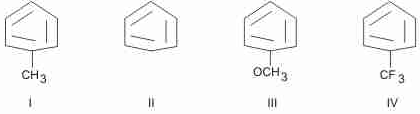Solution:
QUESTION: 33

What will be the product in the following reaction?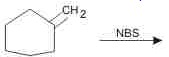Solution:
QUESTION: 34

Given: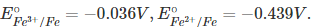The value of standard electrode potential for the change,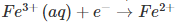(aq) will be

Solution:
QUESTION: 35

'Z' in the following sequence of reactions is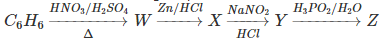Solution:
*Multiple options can be correct
QUESTION: 36

At constant temperature, the osmotic pressure of a solution is

Solution:
*Multiple options can be correct
QUESTION: 37

Which of the following are the examples of aldol condensation ?

Solution:
*Multiple options can be correct
QUESTION: 38

The molecules that will have dipole moment are:

Solution:
*Multiple options can be correct
QUESTION: 39

Keto-enol tautomerism is observed in

Solution:
*Multiple options can be correct
QUESTION: 40

When HCl(g) is passed through a saturated solution of common salt, pure NaCl is precipitated because

Solution:Use Code STAYHOME200 and get INR 200 additional OFF Use Coupon Code

Track your progress, build streaks, highlight & save important lessons and more!

Similar ContentRelated tests